Related Articles

# Angular 7 | Observables

• Last Updated : 25 Sep, 2020

Observables provide support for data sharing between publishers and subscribers in an angular application. It is referred to as a better technique for event handling, asynchronous programming, and handling multiple values as compared to techniques like promises.
A special feature of Observables is that it can only be accessed by a consumer who subscribes to it i.e A function for publishing values is defined, but it is not executed by the subscribed consumer (it can be any component) only via which the customer can receive notifications till the function runs or till they subscribed.
An observable can deliver multiple values of any type. The API for receiving values is the same in any condition and the setup and the logic are both handled by the observable. Rest thing is only about subscribing and unsubscribing the information required.
Observers: To handle receiving observable messages, we need an observable interface which consists of callback methods with respect to the messages by observables. Some of the basic methods are as follows:

• next: It is a handler for each message by observable, it may be called zero or more times after execution starts.
Example: It is a simple example for the usage of next() method of observers.

## javascript

 `import { Component, OnInit } from ``'@angular/core'``;``import {Observable} from ``'rxjs'``;`` ` `@Component({``    ``selector: ``'app-next-example'``,``    ``templateUrl: ``'./next-example.component.html'``,``    ``styleUrls: [``'./next-example.component.css'``]``})` `export class NextExampleComponent implements OnInit {`` ` `    ``constructor() { }`` ` `    ``ngOnInit() {``    ` `        ``// Create a new Observable``        ``const sqnc = ``new` `Observable(countOnetoTen);``     ` `        ``// Execute the Observable and print the``        ``// result of each notification``        ``// next() is a call to countOnetoTen method``        ``// to get the next value from the observable``        ``sqnc.subscribe({``            ``next(num) { console.log(num); }``        ``});``        ` `        ``// This function runs when subscribe()``        ``// is called``        ``function` `countOnetoTen(observer) {``            ` `            ``for``(``var` `i = 1; i <= 10; i++) {``                ` `                ``// Calls the next observable``                ``// notification``                ``observer.next(i);``            ``}``        ` `            ``// Unsubscribe after completing``            ``// the sequence``            ``return` `{unsubscribe(){}};``        ``}``    ``}``}`
• Output: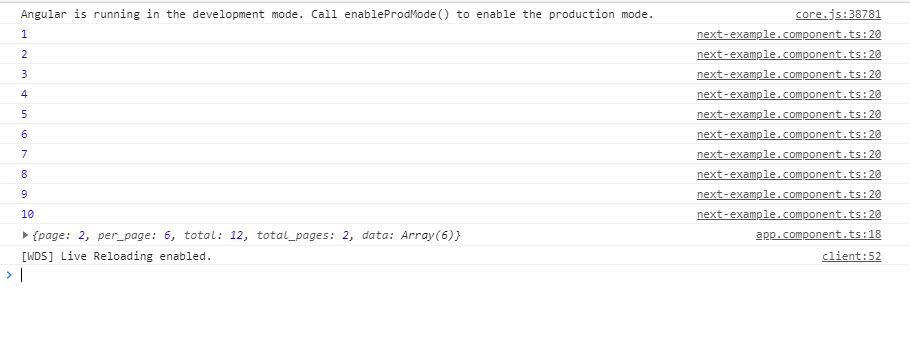•
• error: It is a handler for each error message. An error stops execution of the observable instance.
Example: This is an example, the error is intentionally induced in the code to understand how error works.

## javascript

 `import { Component, OnInit } from ``'@angular/core'``;``import { Observable } from ``'rxjs'``;`` ` `@Component({``    ``selector: ``'app-error-example'``,``    ``templateUrl: ``'./error-example.component.html'``,``    ``styleUrls: [``'./error-example.component.css'``]``})` `export class ErrorExampleComponent implements OnInit {`` ` `    ``constructor() { }`` ` `    ``ngOnInit() {``    ` `        ``// Create a new Observable``        ``const sqnc = ``new` `Observable(generateError);``     ` `        ``// Execute the Observable and print the``        ``// result of each notification``        ``// error() is called when next generate``        ``// some error``        ``sqnc.subscribe({``            ``next(num) { },``            ``error(err) { console.log(``'Error Somewhere'``)}``        ``});``        ` `        ``// This function runs when subscribe() is called``        ``function` `generateError(observer){``            ` `            ``// Calls the next observable notification``            ``// It generates an error and error is called``            ``observer.next( adddlert(``"Welcome guest!"``));``            ` `            ``// Unsubscribe after completing the sequence``            ``return` `{unsubscribe(){}};``        ``}``    ``}`` ` `}`
• Output: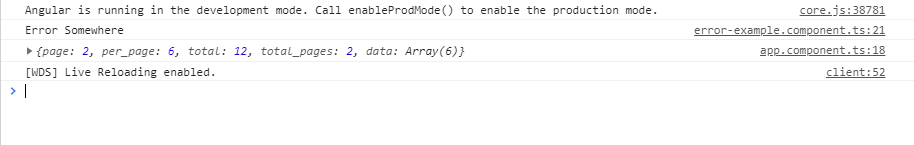• complete: It is a handles in which the completion of observable execution is notified.
Example: This example shows the use of the complete function. The completion notification is triggered by the observer after the completion of the execution of the observable.

## javascript

 `import { Component, OnInit } from ``'@angular/core'``;``import { Observable } from ``'rxjs'``;`` ` `@Component({``    ``selector: ``'app-complete-example'``,``    ``templateUrl: ``'./complete-example.component.html'``,``    ``styleUrls: [``'./complete-example.component.css'``]``})` `export class CompleteExampleComponent implements OnInit {`` ` `    ``constructor() { }`` ` `    ``ngOnInit() {``     ` `        ``// Create a new Observable``        ``const sqnc = ``new` `Observable(countOnetoTen);`` ` `        ``// Execute the Observable and print the``        ``// result of each notification``        ``sqnc.subscribe({``            ``next(num) { console.log(num); },``            ``complete(){console.log(``"Completed!!!!"``)}``        ``});``        ` `        ``// This function runs when subscribe()``        ``// is called``        ``function` `countOnetoTen(observer){``            ` `            ``for``(``var` `i = 1; i <= 10; i++) {``            ` `                ``// Calls the next observable``                ``// notification``                ``observer.next(i);``            ``}``            ` `            ``observer.complete();``            ` `            ``// Unsubscribe after completing``            ``// the sequence``            ``return` `{unsubscribe(){}};``        ``}``    ``}``}`
• Output: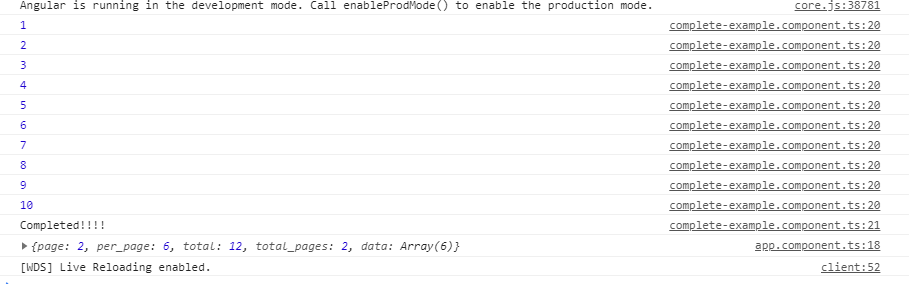•

Making an Observable: In the following example, we will be making a simple observable for getting a table of 2. This code is written in the app.component.ts file. Before using Observables do import Observables from rxjs library by writing the following code.

```import {Observables} from 'rxjs'
```

## javascript

 `import { Component } from ``'@angular/core'``;``import {Observable} from ``"rxjs"``;``import { CompileTemplateMetadata } from ``'@angular/compiler'``;`` ` `@Component({``    ``selector: ``'app-rt'``,``    ``templateUrl: ``'./app.component.html'``,``    ``styleUrls: [``'./app.component.css'``]``})` `export class AppComponent {``    ``title = ``'MyFirstApp'``;``}` `// Create a new Observable that will``// deliver the above sequence``const table = ``new` `Observable(tableOfTwo);`` ` `// Execute the Observable and print the``// result of each notification``// next() is a call to tableOfTwo() method``// to get the next value from the observable``table.subscribe({``    ``next(num) { console.log(num); },``    ``complete() { console.log(``'Finished sequence'``); }``});` `// This function runs when subscribe() is called``function` `tableOfTwo(observer) {``    ` `    ``for``(``var` `i = 1; i <= 10; i++) {``      ``observer.next(``'2 * '` `+ i + ``' = '` `+ i*2);``    ``}``    ` `    ``observer.complete();``    ``return` `{unsubscribe(){}};``}`

In this code, the next() is used to call the next return form the observable and in the observable, after the task is completed it returns unsubscribe function which leads to unsubscription of the observable and thus no more request can be undertaken. When the complete() method is called then it prints the string ‘Finished sequence’. All the functionality are been shown in the console.
Output: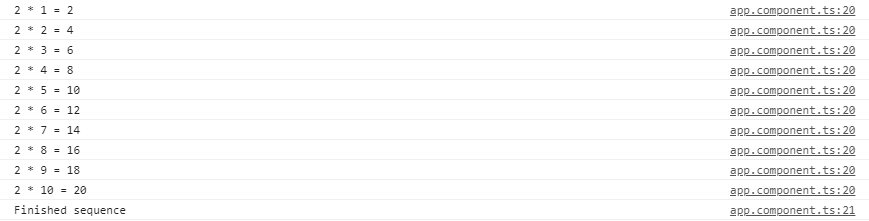Multicasting: It is a practice of broadcasting an observable to a list of multiple subscribers in a single execution. With multicasting observable, there are no multiple listeners to be registered on the document, but instead, the first listener is reused and the values are sent out to each subscriber. It is done by determining the observable about whether or not values are to be multicasted.
Continuing with the above example, now multicast operation will be performed, which will cast the same table of two on two 2 sequences and will wait for a second before doing another operation.

## javascript

 `import { Component, OnInit } from ``'@angular/core'``;``import {Observable} from ``'rxjs'``;`` ` `@Component({``    ``selector: ``'app-my-page'``,``    ``templateUrl: ``'./my-page.component.html'``,``    ``styleUrls: [``'./my-page.component.css'``]``})` `export class MyPageComponent implements OnInit {`` ` `    ``constructor() { }`` ` `    ``ngOnInit() {``    ` `        ``const multiSeq = ``new` `Observable(``this``.multiSeqSubs());``   ` ` ` `        ``multiSeq.subscribe({``            ``next(num) { console.log(``'1st subscribe: '` `+ num); },``            ``complete() { console.log(``'1st sequence finished.'``); }``        ``});``     ` `        ``// Subscribe again After 1 seconds.``        ``setTimeout(() => {``            ``multiSeq.subscribe({``                ``next(num) { console.log(``'2nd subscribe: '` `+ num); },``                ``complete() { console.log(``'2nd sequence finished.'``); }``            ``});``        ``}, 1000);``    ``}`` ` `    ``multiSeqSubs() {``        ``const seq = [];``        ` `        ``for` `(``var` `i = 1; i <= 10; i++) {``            ` `            ``// Pushes the string onto sequence``            ``seq.push(``'2 * '` `+ i + ``'='` `+ 2*i)``        ``}  ``    ` `        ``// Keep track of each observer``        ``const obs = [];``        ` `        ``// A single time Stamp for one``        ``// set of values being generated,``        ``// multicasted to each subscriber``        ``let timeStamp;``   ` `        ``// Return the subscriber function``        ``// (runs when subscribe() function``        ``// is invoked)``        ``return` `(ob) => {``            ``obs.push(ob);``            ` `            ``// When this is the first subscription,``            ``// start the sequence``            ``if` `(obs.length === 1) {``                ``timeStamp = ``this``.exec_Sequence({``                    ``next(val) {``                        ` `                        ``// Iterate through observers``                        ``// and notify all subscriptions``                        ``obs.forEach(o => o.next(val));``                    ``},``                    ` `                    ``complete() {``                        ` `                        ``// Notify all complete callbacks``                        ``obs.slice(0).forEach(o => o.complete());``                    ``}``                ``}, seq, 0);``            ``}``   ` `            ``return` `{``                ` `                ``// Unsubscribe from the observers``                ``unsubscribe() {``                ` `                    ``obs.splice(obs.indexOf(ob), 1);``                    ` `                    ``// Cleanup``                    ``if` `(obs.length === 0) {``                        ``clearTimeout(timeStamp);``                    ``}``                ``}``            ``};``        ``};``    ``}``   ` `    ``// Executes the sequence``    ``exec_Sequence(observer, sequence, index) {``        ``return` `setTimeout(() => {``            ``observer.next(sequence[index]);``            ``if` `(index === sequence.length - 1) {``                ``observer.complete();``            ``} ``else` `{``                ``this``.exec_Sequence(observer, sequence, ++index);``            ``}``        ``}, 1000);``    ``}``   ` `    ``// Create a new Observable that will``    ``// deliver the above sequence``}`

This code is doing the same functionality i.e. handling multicast operations. In this code, we have a list of observers that depends on the no. of subscriptions made for the multicast operation. Here during the code execution, we have only 2 operations that are executing and hence we have only 2 elements in the ‘obs’ list.
Output: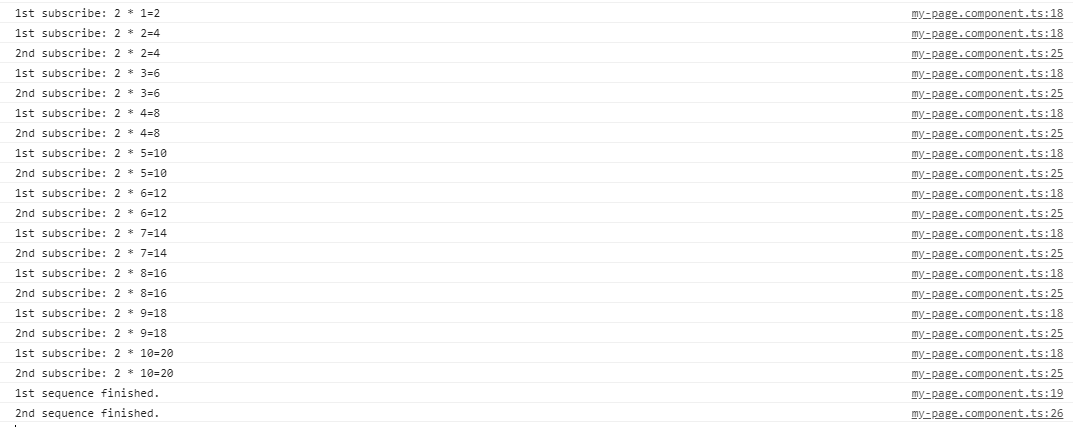Error Handling:
Observables produce asynchronous values and thus try/catch do not catch any errors because it may lead to stop the code irrespective of other tasks running at that instance of time. Instead, we handle errors by specifying an error callback on the observer. When an error is produced, it causes the observable to clean up subscriptions and stop producing values for that subscription. An observable can either produce values (calling the next callback), or it can complete, calling either the complete or error callback.

The syntax for error callback

```observable.subscribe({
next(val) { console.log('Next: ' + val)},
error(err) { console.log('Error: ' + err)}
});

```

My Personal Notes arrow_drop_up